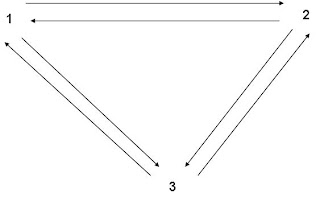## M Theory Lesson 13

John Baez’s TWF244 is really cool. He talks about a recent paper by Leinster on the Euler characteristic of a finite category. Consider the following. Order the objects in the category $1, 2, 3, \cdots ,n$. The integral adjacency matrix for the category sets $a_{ij}$ to be the number of arrows from $i$ to $j$.

For example, in the categorywe would have a 3×3 matrix with all entries 1, since we must not forget the identity arrows. If the inverse to this matrix $A$ existed, the Euler characteristic would be the sum of entries in $A^{-1}$. Let’s fill in a few more arrows. Imagine there were $k$ arrows from $1$ to $2$, $2$ to $3$ and $1$ to $3$ which forms a basic composition triangle. Similarly, imagine the dual triangle had $m$ arrows at each edge. Then the adjacency matrix would be a circulant based on $1,k$ and $m$. Such triangles look like idempotent equations $k^2 = k$, but here $k$ is an ordinal and we should wonder about composing one of the $k$ arrows from $1$ to $2$ with another one from $2$ to $3$, because the number of such compositions would naively be more than $k$. If $k^2 = k$ were true, and $k$ was ordinal, then it must be zero or one, which gives a particularly simple kind of category otherwise known as a poset.

Anyway, to cut a long story short, this characteristic works nicely for all sorts of things, such as orbifolds. In M Theory we counted the number of particle generations using an orbifold Euler characteristic, which might be a rational number in general. So we can think of this as a cardinality of a category! This is wonderful, because the physical result follows from the universality of $\chi$.

Moerdijk looked at Lie groupoids as a foundation for orbifolds, which seems like a logical thing to do from the Symmetry point of view. But remember that we encountered the orbifold Euler characteristic in the work of Mulase et al on ribbon matrix models.

## 6 Responses so far »

1. 1### L. Riofrio said,

These diagrams look similiar to the Eightfold Way. That makes one wonder whether non-linear operads will be key to extending the Standard Model. That would be refreshing, since physics has gone nowhere in 30 years.

2. 2### Kea said,

Yeah. And the ribbon diagrams are just what ‘t Hooft ordered for QCD back in 1974. Seems so simple, doesn’t it?

3. 3### Doug said,

Hi Kea

Thanks for the link to TWF 244. As usual John Baez has a great and interesting blog.

I am fascinated with item 5 which discusses Euler’s bridges. From my perspective this is a problem ideal for the strategy analysis of game theory.

Geert Jan Olsder [mathematics] Delft University in 2005 presented a variant of this problem as a train routing schedule using game theory.
MAX PLUS IN HET (TREIN)VERKEER …[Text in English]
webserv.nhl.nl/~kamminga/wintersymposium/Olsder2005.pdf

If you like, on Amazon.com you can view inside this great book: Dynamic Noncooperative Game Theory (Classics in Applied Mathematics) (Paperback)
by Tamer Basar, Geert Jan Olsder.

Basar is also still active as a Professor of Electrical and Computer Engineering. Engineers might be considered “applied physicists“.

4. 4### Kea said,

Doug, we appreciate your enthusiasm for Game Theory, but I have to say, I’m not sure when I’m going to find time to read any of these references, once I slog through a few papers in particle physics, math phys, maths, cosmology, philosophy ….. sigh. So little time.

5. 5### kneemo said,

Thanks for the Leinster reference Kea. I’m pleased to know that whatever the fundamental objects of M-theory are, we can still talk about their Euler characteristic!

6. 6### Kea said,

Why, yes, kneemo! This is important. We can’t go to all this trouble to create a ‘true BI’ theory and then throw it all out by cheating on the actual number crunching at the end. The numbers must fall out of a universal construction.